# Drawing shapes¶

OpenCV has different drawing functions to draw:

• lines
• circle
• rectangle
• ellipse
• text

## Using Numpy¶

Numpy is a very powerful math module for dealing with multi-dimensional data such as vectors and images. The OpenCV images are represented as Numpy arrays. At the start of a program we import both:

```import cv2 as cv
import numpy as np
```

To create an empty color image we create a 3D array of zeroes:

```img = img = np.zeros((100, 600, 3), np.uint8)
cv.imshow('RGB', img)
```

When zooming, we can see the 3 color components.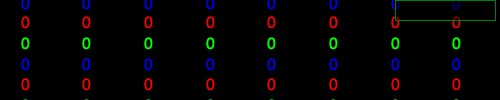To create an empty gray-scale image we create a 2D array of zeroes:

```gray_img = np.zeros((100, 600), np.uint8)
cv.imshow('Gray', gray_img)
```

The grayscale values for each pixel go from 0 to 255. In a black image all pixel values are 0.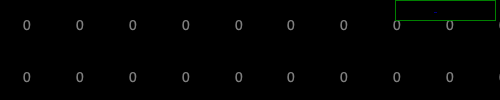```import cv2 as cv
import numpy as np

img = img = np.zeros((100, 500, 3), np.uint8)
cv.imshow('RGB', img)

gray_img = np.zeros((100, 500), np.uint8)
cv.imshow('Gray', gray_img)

cv.waitKey(0)
cv.destroyAllWindows()
```

`draw1.py`

## Define colors¶

Colors are defined by three base colors: Blue, Green and Red. All three put to zero gives black, all three at the maximum gives white:

```BLACK = (0, 0, 0)
WHITE = (255, 255, 255)
```

Different from the more common RGB ordering, OpenCV uses the ordering BGR:

```RED = (0, 0, 255)
GREEN = (0, 255, 0)
BLUE = (255, 0, 0)
```

Mixing color components results in more colors:

```CYAN = (255, 255, 0)
MAGENTA = (255, 0, 255)
YELLOW = (0, 255, 255)
```

## Draw a line¶

The function `cv.line()` adds a line to an image:

```cv.line(image, p0, p1, color, thickness)
```
• image where the line is added
• start point p0
• end point p1
• line color
• line thickness

Lets define three points:

```p0 = 10, 10
p1 = 300, 90
p2 = 500, 10
```

Now we can draw two colored lines:

```cv.line(img, p0, p1, RED, 2)
cv.line(img, p1, p2, YELLOW, 5)
```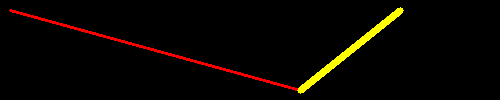If the image is a gray-scale image, instead of the color triplet, a grayscale value from 0 (black) to 255 (white) is used:

```cv.line(gray_img, p0, p1, 100, 2)
cv.line(gray_img, p1, p2, 255,5)
```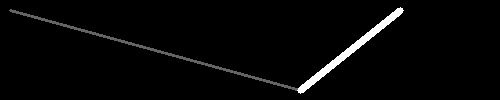```import cv2 as cv
import numpy as np

RED = (0, 0, 255)
YELLOW = (0, 255, 255)

p0, p1, p2 = (10, 10), (300, 90), (400, 10)

img = img = np.zeros((100, 500, 3), np.uint8)
cv.line(img, p0, p1, RED, 2)
cv.line(img, p1, p2, YELLOW, 5)
cv.imshow('RGB', img)

gray_img = np.zeros((100, 500), np.uint8)
cv.line(gray_img, p0, p1, 100, 2)
cv.line(gray_img, p1, p2, 255,5)
cv.imshow('Gray', gray_img)

cv.waitKey(0)
cv.destroyAllWindows()
```

`line1.py`

## Select thickness with a trackbar¶

We can use a trackbar to set the thickness of the line. The trackbar callback function has one argument - the line thickness. Trackbar arguments are always integer and they always start at 0. However, for lines the smallest line thickness is 1. Therefore we use the `max` function to make the thickness at least 1. To have a numeric feedback, we display the thickness value also in the overlay:

```def trackbar(x):
x = max(1, x)
cv.displayOverlay('window', f'thickness={x}')
```

Next we have to redraw the line. We start by resetting the image to 0. Then we draw the line and display the new image:

```img[:] = 0
cv.line(img, p0, p1, RED, x)
cv.imshow('window', img)
```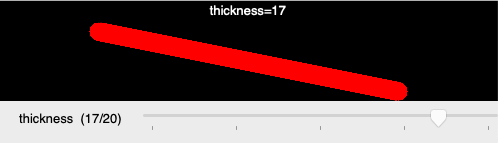```# Select line thickness with a trackbar
import cv2 as cv
import numpy as np

RED = (0, 0, 255)
p0, p1 = (100, 30), (400, 90)

def trackbar(x):
x = max(1, x)
cv.displayOverlay('window', f'thickness={x}')
img[:] = 0
cv.line(img, p0, p1, RED, x)
cv.imshow('window', img)

img = np.zeros((100, 500, 3), np.uint8)
cv.line(img, p0, p1, RED, 2)
cv.imshow('window', img)
cv.createTrackbar('thickness', 'window', 2, 20, trackbar)

cv.waitKey(0)
cv.destroyAllWindows()
```

`line2.py`

## Select color with a trackbar¶

We can use a trackbar to set the line color. The trackbar is used to select an index into a color list which we define with 7 colors:

```colors = (RED, GREEN, BLUE, CYAN, MAGENTA, YELLOW, WHITE)
```

The trackbar is defined to return an integer value from 0 to 6:

```cv.createTrackbar('color', 'window', 0, 6, trackbar)
```

Inside the trackbar callback function we use the index to look up the color. To give numeric feedback we display the color RGB value in the overlay:

```def trackbar(x):
color = colors[x]
cv.displayOverlay('window', f'color={color}')
```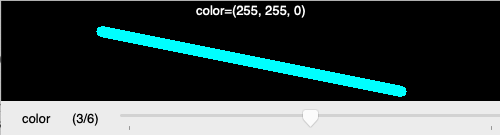```# Select line color with a trackbar
import cv2 as cv
import numpy as np

RED = (0, 0, 255)
GREEN = (0, 255, 0)
BLUE = (255, 0, 0)
CYAN = (255, 255, 0)
MAGENTA = (255, 0, 255)
YELLOW = (0, 255, 255)
WHITE = (255, 255, 255)

colors = (RED, GREEN, BLUE, CYAN, MAGENTA, YELLOW, WHITE)
p0, p1 = (100, 30), (400, 90)

def trackbar(x):
color = colors[x]
cv.displayOverlay('window', f'color={color}')
img[:] = 0
cv.line(img, p0, p1, color, 10)
cv.imshow('window', img)

img = np.zeros((100, 500, 3), np.uint8)
cv.line(img, p0, p1, RED, 10)
cv.imshow('window', img)
cv.createTrackbar('color', 'window', 0, 6, trackbar)

cv.waitKey(0)
cv.destroyAllWindows()
```

`line3.py`

## Select end point with the mouse¶

Let’s use the mouse for selecting the end point of the line. The mouse callback function has the x and y coordinates as arguments. We are only interesed in the mouse movement when a button is pressed (flags==1). The current mouse coordinates will be the new end point for the line. We display this coordinates in the overlay:

```def mouse(event, x, y, flags, param):
if flags == 1:
p1 = x, y
cv.displayOverlay('window', f'p1=({x}, {y})')
```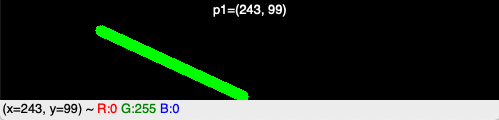```# Select end point with the mouse
import cv2 as cv
import numpy as np

GREEN = (0, 255, 0)
p0, p1 = (100, 30), (400, 90)

def mouse(event, x, y, flags, param):
if flags == 1:
p1 = x, y
cv.displayOverlay('window', f'p1=({x}, {y})')
img[:] = 0
cv.line(img, p0, p1, GREEN, 10)
cv.imshow('window', img)

img = np.zeros((100, 500, 3), np.uint8)
cv.line(img, p0, p1, GREEN, 10)
cv.imshow('window', img)
cv.setMouseCallback('window', mouse)

cv.waitKey(0)
cv.destroyAllWindows()
```

`line4.py`

## Draw a complete line¶

Let’s now draw a complete line with the mouse. Now we need to distinguish between mouse down, mouse move and mouse up events. When the mouse is down, we start a new line and set both points `p0` and `p1` to the current mouse position:

```if event == cv.EVENT_LBUTTONDOWN:
p0 = x, y
p1 = x, y
```

If the mouse moves (with the button pressed) or if the mouse button goes up, we only set `p1` to the new mouse position:

```elif event == cv.EVENT_MOUSEMOVE and flags == 1:
p1 = x, y

elif event == cv.EVENT_LBUTTONUP:
p1 = x, y
```

At the end of the mouse callback function we reset the image to zero (black), draw the line, display the new image and show the two points in the overlay:

```img[:] = 0
cv.line(img, p0, p1, RED, 10)
cv.imshow('window', img)
cv.displayOverlay('window', f'p0={p0}, p1={p1}')
```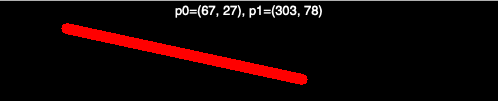```# Draw a complete line with the mouse
import cv2 as cv
import numpy as np

RED = (0, 0, 255)
p0, p1 = (100, 30), (400, 90)

def mouse(event, x, y, flags, param):
global p0, p1

if event == cv.EVENT_LBUTTONDOWN:
p0 = x, y
p1 = x, y

elif event == cv.EVENT_MOUSEMOVE and flags == 1:
p1 = x, y

elif event == cv.EVENT_LBUTTONUP:
p1 = x, y

img[:] = 0
cv.line(img, p0, p1, RED, 10)
cv.imshow('window', img)
cv.displayOverlay('window', f'p0={p0}, p1={p1}')

img = np.zeros((100, 500, 3), np.uint8)
cv.imshow('window', img)
cv.setMouseCallback('window', mouse)

cv.waitKey(0)
cv.destroyAllWindows()
```

`line5.py`

## Draw multiple lines¶

How do we do to draw multiple lines to an image? First we need to have a temporary copy `img0` which contains the lines of the previous stage of the drawing:

```img0 = np.zeros((100, 500, 3), np.uint8)
img = img0.copy()
```

When the mouse button is down, we set the two points `p0` and `p1` to the current mouse position:

```if event == cv.EVENT_LBUTTONDOWN:
p0 = x, y
p1 = x, y
```

When the mouse moves, we reset the current image to the previous image `img0` and draw a blue line of thickness 2:

```elif event == cv.EVENT_MOUSEMOVE and flags == 1:
p1 = x, y
img[:] = img0
cv.line(img, p0, p1, BLUE, 2)
```

When the mouse goes up, we reset the image to the previous image `img0`, draw a red line of thickness 4, and save this new image as `img0`:

```elif event == cv.EVENT_LBUTTONUP:
img[:] = img0
cv.line(img, p0, p1, RED, 4)
img0[:] = img
```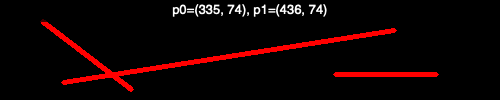```# Draw multiple lines with the mouse
import cv2 as cv
import numpy as np

RED = (0, 0, 255)
BLUE = (255, 0, 0)
p0, p1 = (100, 30), (400, 90)

def mouse(event, x, y, flags, param):
global p0, p1

if event == cv.EVENT_LBUTTONDOWN:
p0 = x, y
p1 = x, y

elif event == cv.EVENT_MOUSEMOVE and flags == 1:
p1 = x, y
img[:] = img0
cv.line(img, p0, p1, BLUE, 2)

elif event == cv.EVENT_LBUTTONUP:
img[:] = img0
cv.line(img, p0, p1, RED, 4)
img0[:] = img

cv.imshow('window', img)
cv.displayOverlay('window', f'p0={p0}, p1={p1}')

img0 = np.zeros((100, 500, 3), np.uint8)
img = img0.copy()
cv.imshow('window', img)
cv.setMouseCallback('window', mouse)

cv.waitKey(0)
cv.destroyAllWindows()
```

`line6.py`

## Draw a rectangle¶

The function `cv.rectangle()` adds a rectangle to an image:

```cv.rectangle(image, p0, p1, color, thickness)
```
• image where the rectangle is added
• corner point p0
• corner point p1
• ouline color
• line thickness

If the line thickness is negative or `cv.FILLED` the rectangle is filled:

```cv.rectangle(img, p0, p1, BLUE, 2)
cv.rectangle(img, p2, p3, GREEN, cv.FILLED)
```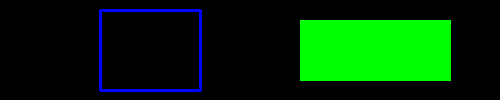```import cv2 as cv
import numpy as np

BLACK = (0, 0, 0)
WHITE = (255, 255, 255)

RED = (0, 0, 255)
GREEN = (0, 255, 0)
BLUE = (255, 0, 0)

CYAN = (255, 255, 0)
MAGENTA = (255, 0, 255)
YELLOW = (0, 255, 255)

p0 = 100, 10
p1 = 200, 90
p2 = 300, 20
p3 = 450, 80

img = img = np.zeros((100, 500, 3), np.uint8)
cv.rectangle(img, p0, p1, BLUE, 2)
cv.rectangle(img, p2, p3, GREEN, cv.FILLED)
cv.imshow('RGB', img)

cv.waitKey(0)
cv.destroyAllWindows()
```

`rect1.py`

## Draw multiple rectangles¶

Now we combine thickness and color trackbar as welle as the mouse callback to create multple rectangles.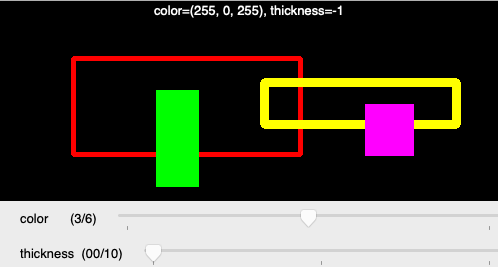```import cv2 as cv
import numpy as np
from draw import *

def draw(x):
global p0, p1
d = cv.getTrackbarPos('thickness', 'window')
d = -1 if d==0 else d
i = cv.getTrackbarPos('color', 'window')
color = colors[i]
img[:] = img0
cv.rectangle(img, p0, p1, color, d)
cv.imshow('window', img)
text = f'color={color}, thickness={d}'
cv.displayOverlay('window', text)

def mouse(event, x, y, flags, param):
global p0, p1
if event == cv.EVENT_LBUTTONDOWN:
img0[:] = img
p0 = x, y
elif event == cv.EVENT_MOUSEMOVE and flags == 1:
p1 = x, y
elif event == cv.EVENT_LBUTTONUP:
p1 = x, y
draw(0)

cv.setMouseCallback('window', mouse)
cv.createTrackbar('color', 'window', 0, 6, draw)
cv.createTrackbar('thickness', 'window', 0, 10, draw)

cv.waitKey(0)
cv.destroyAllWindows()
```

`rect2.py`

The common code such as color definitions and image creation has been placed in a separate file.

```import numpy as np
import cv2 as cv

BLACK = (0, 0, 0)
WHITE = (255, 255, 255)

RED = (0, 0, 255)
GREEN = (0, 255, 0)
BLUE = (255, 0, 0)

CYAN = (255, 255, 0)
MAGENTA = (255, 0, 255)
YELLOW = (0, 255, 255)

colors = (RED, GREEN, BLUE, MAGENTA, CYAN, YELLOW, WHITE)
p0 = p1 = 0, 0

img0 = np.zeros((200, 500, 3), np.uint8)
img = img0.copy()
cv.imshow('window', img)
```

`draw.py`

## Draw an ellipse¶

The function `cv.ellipes()` adds an ellipse to an image:

```cv.ellipse(img, center, axes, angle, a0, a1, color, thickness)
```
• image where the ellipse is added
• center point
• the two axes
• the axis orientation angle
• the beginning angle a0
• the ending angle a1
• ouline color
• line thickness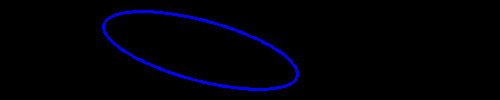```import cv2 as cv
import numpy as np

BLUE = (255, 0, 0)
center = 200, 50
axes = 100, 30
angle = 15

img = img = np.zeros((100, 500, 3), np.uint8)
cv.ellipse(img, center, axes, angle, 0, 360, BLUE, 2)
cv.imshow('RGB', img)

cv.waitKey(0)
cv.destroyAllWindows()
```

`draw4.py`

## Draw a polygon¶

The `polylines` function expects a Numpy array for the point list:

```pts = [(50, 50), (300, 190), (400, 10)]
cv.polylines(img, np.array([pts]), True, RED, 5)
```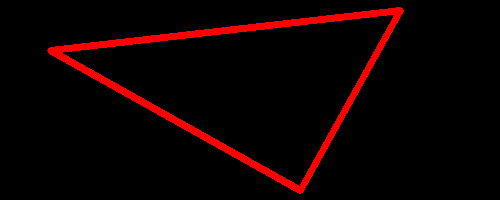```import cv2 as cv
import numpy as np

RED = (0, 0, 255)
pts = [(50, 50), (300, 190), (400, 10)]

img = img = np.zeros((200, 500, 3), np.uint8)
cv.polylines(img, np.array([pts]), True, RED, 5)
cv.imshow('window', img)

cv.waitKey(0)
cv.destroyAllWindows()
```

`polygon1.py`

## Draw a filled polygon¶

The `polylines` function expects a Numpy array for the point list:

```pts = [(50, 50), (300, 190), (400, 10)]
cv.polylines(img, np.array([pts]), True, RED, 5)
```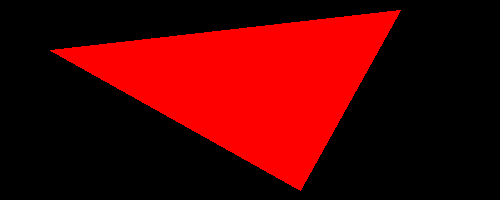```import cv2 as cv
import numpy as np

RED = (0, 0, 255)
pts = [(50, 50), (300, 190), (400, 10)]

img = img = np.zeros((200, 500, 3), np.uint8)
cv.fillPoly(img, np.array([pts]), RED)
cv.imshow('window', img)

cv.waitKey(0)
cv.destroyAllWindows()
```

`polygon2.py`

## Draw a polygon with the mouse¶

Combining the previous techniques, it is rather simple to draw a polygon just by clicking into the window. First we define an empty list:

```pts = []
```

Each time we click with the mouse we append a point:

```def mouse(event, x, y, flags, param):
if event == cv.EVENT_LBUTTONDOWN:
pts.append((x, y))
draw(0)
```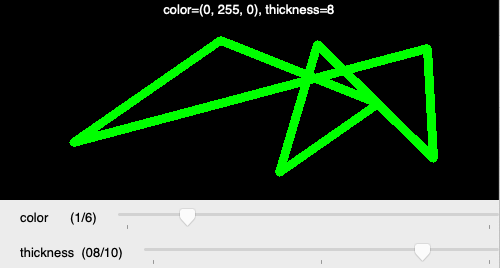```import cv2 as cv
import numpy as np
from draw import *

pts = []

def draw(x):
d = cv.getTrackbarPos('thickness', 'window')
d = -1 if d==0 else d
i = cv.getTrackbarPos('color', 'window')
color = colors[i]
img[:] = img0
cv.polylines(img, np.array([pts]), True, color, d)
cv.imshow('window', img)
text = f'color={color}, thickness={d}'
cv.displayOverlay('window', text)

def mouse(event, x, y, flags, param):
if event == cv.EVENT_LBUTTONDOWN:
pts.append((x, y))
draw(0)

cv.setMouseCallback('window', mouse)
cv.createTrackbar('color', 'window', 0, 6, draw)
cv.createTrackbar('thickness', 'window', 2, 10, draw)
draw(0)

cv.waitKey(0)
cv.destroyAllWindows()
```

`polygon3.py`

## Draw text¶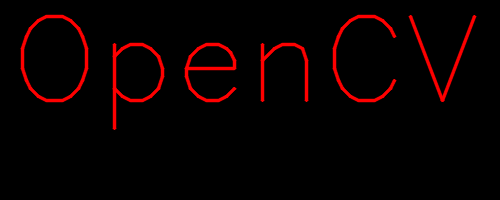```import cv2 as cv
import numpy as np

RED = (0, 0, 255)
p0 = (10, 100)

font = cv.FONT_HERSHEY_SIMPLEX
img = np.zeros((200, 500, 3), np.uint8)
cv.putText(img,'OpenCV', p0, font, 4, RED, 2, cv.LINE_AA)
cv.imshow('window', img)

cv.waitKey(0)
cv.destroyAllWindows()
```

`text1.py`